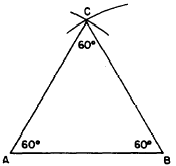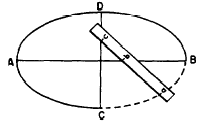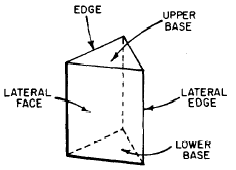Custom SearchFINDING THE CENTER OF A CIRCLE It is sometimes necessary to find the center of a circle of which only an arc or a segment is given. (See fig. 18-8.) From any point on the am, such as A, draw two chords intersecting the arc in any two Points, such as B and C. With the points A, B, and C as centers, use any convenient radius and draw short intersecting arcs to form the perpendicular bisectors of chords AC and AB. Join the intersecting arcs on each side of AC, obtaining line MP, and join the arcs on each side of AB, obtaining line NQ. The intersection of MP and NQ is point 0, the center of the circle. ELLIPSES An ellipse of specified length and width is constructed as follows: 1. Draw the major axis, AB, and the minor axis, CD, as shown in figure 18-9. 2. On a straightedge or ruler, mark a point (labeled a in the figure) and from this point measure one-half the length of the minor axis and make a second mark (b in figure 18-9). From point a, measure one-half the length ofFigure 18-6. - Constructing a right angle by the 3-4-5 method.Figure 18-7 - Constructing 60° angles.Figure 18-8 - Finding the center of a circle.Figure 18-9.-Constructing an ellipse. the major axis and make a third mark (c in the figure). 3. Place the straightedge on the axes so that b lies on the major axis and c lies on the minor axis. Mark the paper with a dot beside point a. Reposition the straightedge, keeping b on the major axis and c on the minor axis, and make a dot beside the new position of a. 4. After locating enough dots to see the elliptical pattern, join the dots with a smooth curve. SOLID FIGURES The plane figures discussed in chapter 17 of this course are combined to form solid figures. For example, three rectangles and two triangles may be combined as shown in figure 18-10. The flat surfaces of the solid figure are its FACES; the top and bottom faces are the BASES, and the faces forming the sides are the LATERAL FACES.Figure 18-10.-Parts of a solid figure. Some solid figures do not have any flat faces, and some have a combination of curved surfaces and flat surfaces. Examples of solids with curved surfaces include cylinders, cones, and spheres.